Next: Stability of Lagrange points Up: Three-body problem Previous: Lagrange points

# Zero-velocity surfaces

Consider the surface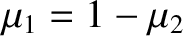(9.60)

where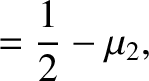(9.61)

Note that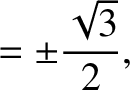. It follows, from Equation (9.35), that if the mass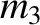has the Jacobi integral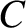and lies on the surface specified in Equation (9.60), then it must have zero velocity. Hence, such a surface is termed a zero-velocity surface. The zero-velocity surfaces are important because they form the boundary of regions from which the massis dynamically excluded; that is, regions where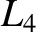. Generally speaking, the regions from whichis excluded grow in area asincreases, and vice versa.

Let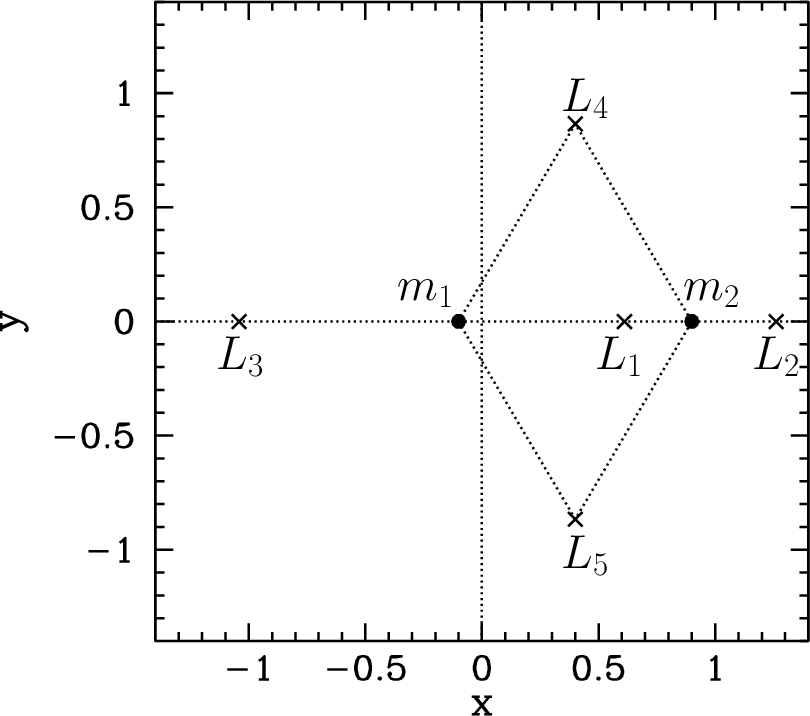be the value of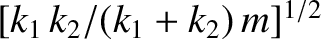at the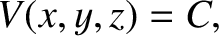Lagrange point, for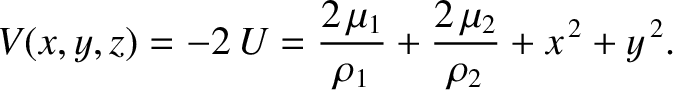. When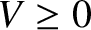, it is easily demonstrated that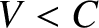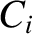(9.62)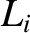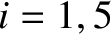(9.63)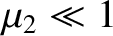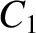(9.64)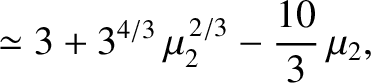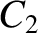(9.65) and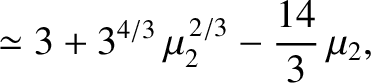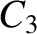(9.66)

Note that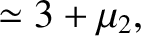.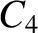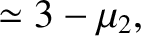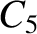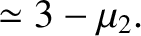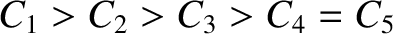Figures 9.7 through 9.11 show the intersection of the zero-velocity surface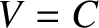with the-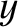plane for various different values of, and illustrate how the region from whichis dynamically excluded--which we shall term the excluded region--evolves as the value ofis varied. Of course, any point not in the excluded region is in the so-called allowed region. For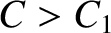, the allowed region consists of two separate oval regions centered on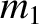and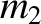, respectively, plus an outer region that lies beyond a large circle centered on the origin. All three allowed regions are separated from one another by an excluded region. (See Figure 9.7.) When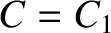, the two inner allowed regions merge at the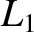point. (See Figure 9.8.) When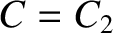, the inner and outer allowed regions merge at the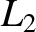point, forming a horseshoe-like excluded region. (See Figure 9.9.) When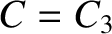, the excluded region splits in two at the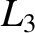point. (See Figure 9.10.) For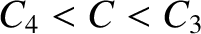, the two excluded regions are localized about the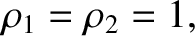and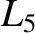points. (See Figure 9.11.) Finally, for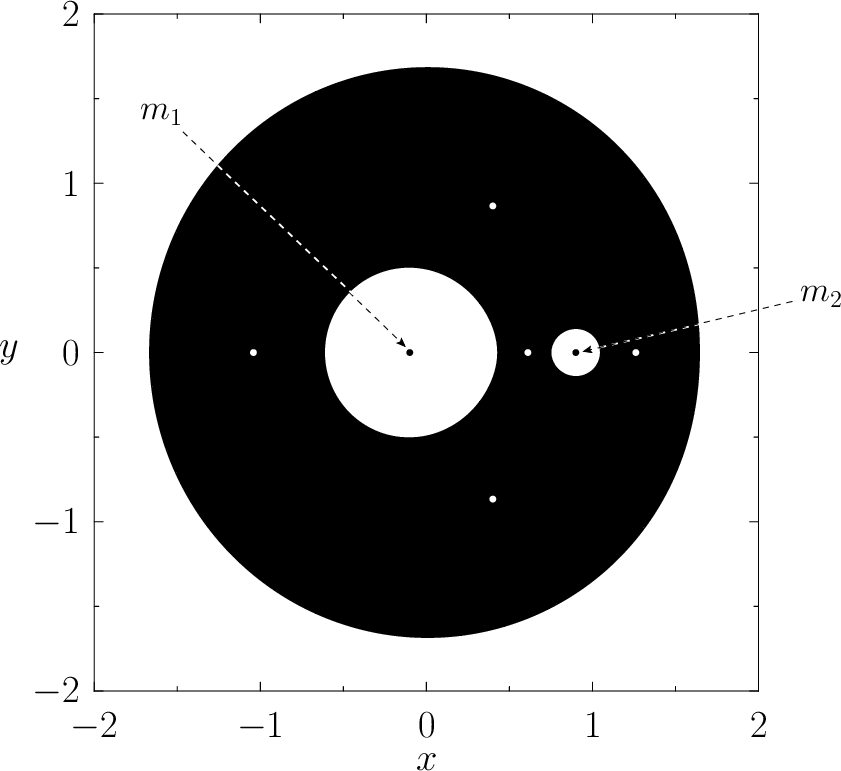, there is no excluded region.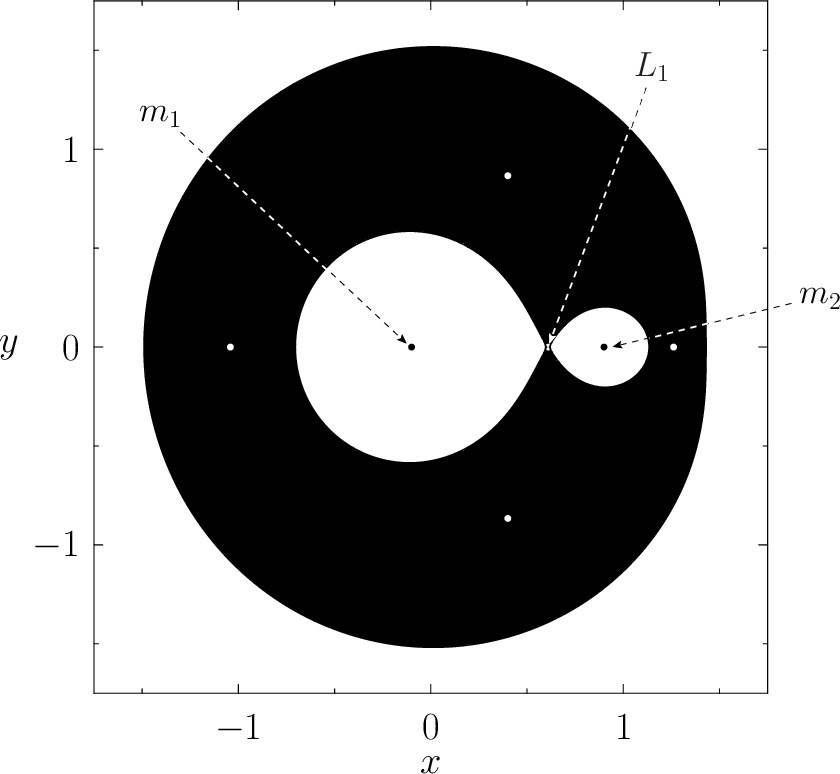Figure 9.12 shows the zero-velocity surfaces and Lagrange points calculated for the case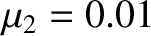. It can be seen that, at very small values of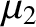, theandLagrange points are almost equidistant from mass. Furthermore, mass, and the,, andLagrange points all lie approximately on a unit circle, centered on mass. It follows that, whenis small, the Lagrange points,andall share the orbit of massabout(in the inertial frame) with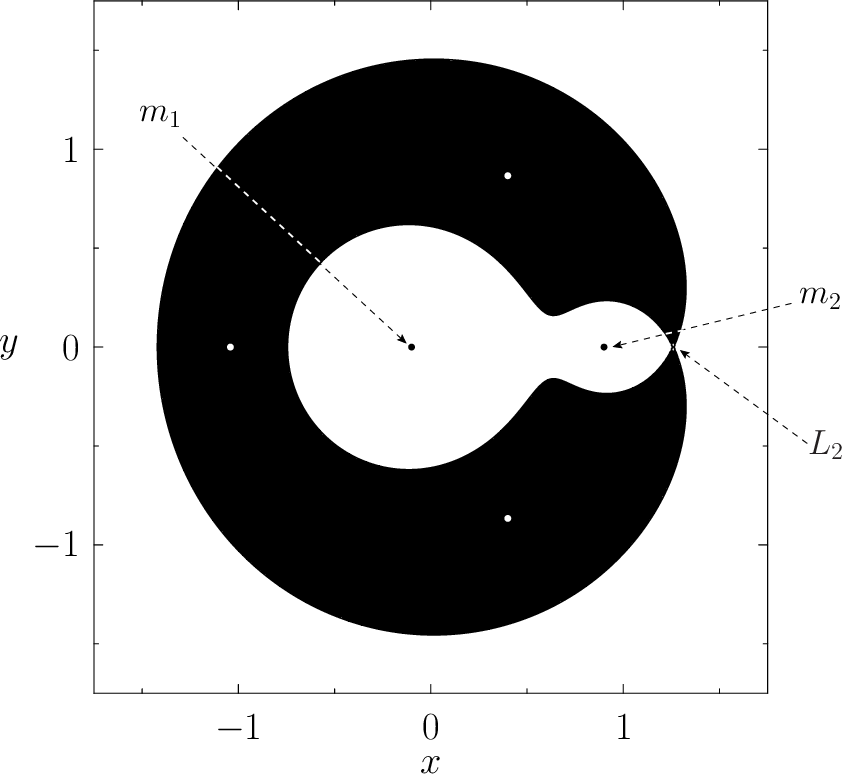being directly opposite,(by convention)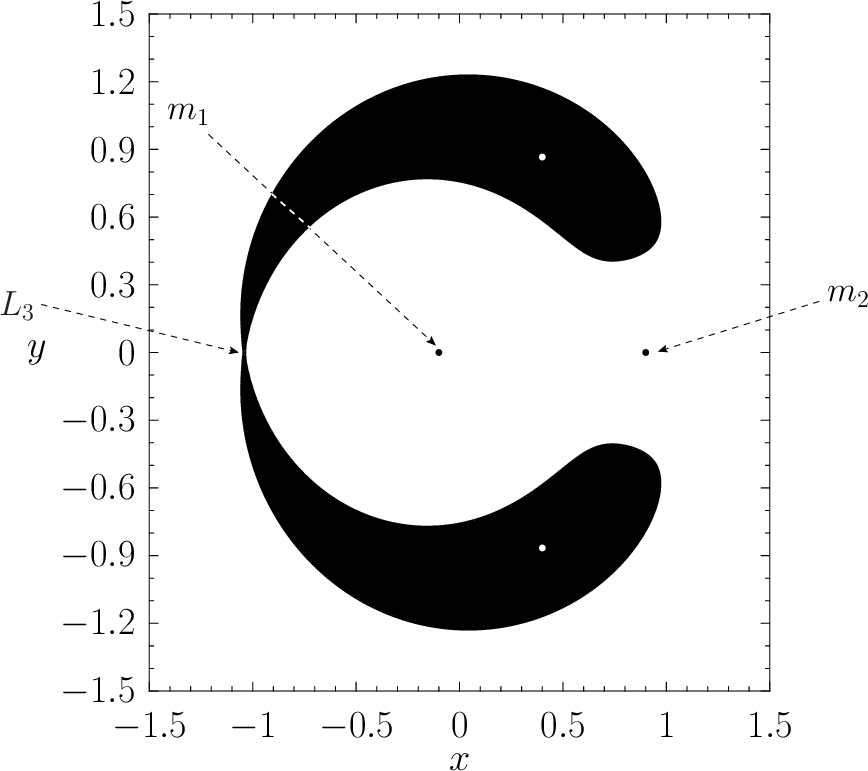ahead of, andbehind.Next: Stability of Lagrange points Up: Three-body problem Previous: Lagrange points
Richard Fitzpatrick 2016-03-31